# Data Exploration with Pandas

## Exploratory analysis in Python using Pandas

In order to explore our data further, let me introduce you to another animal (as if Python was not enough!) – PandasPandas is one of the most useful data analysis library in Python (I know these names sounds weird, but hang on!). They have been instrumental in increasing the use of Python in data science community. We will now use Pandas to read a data set from a well known online competition, perform exploratory analysis and build our first basic categorization algorithm for solving this problem.

Before loading the data, lets understand the 2 key data structures in Pandas – Series and DataFrames

### Introduction to Series and Dataframes

Series can be understood as a 1 dimensional labelled / indexed array. You can access individual elements of this series through these labels.

A dataframe is similar to Excel workbook – you have column names referring to columns and you have rows, which can be accessed with use of row numbers. The essential difference being that column names and row numbers are known as column and row index, in case of dataframes.

Series and dataframes form the core data model for Pandas in Python. The data sets are first read into these dataframes and then various operations (e.g. group by, aggregation etc.) can be applied very easily to its columns.

More: 10 Minutes to Pandas

### Practice data set – Loan Prediction Problem

The dataset here, has the description of variables as:

```VARIABLE DESCRIPTIONS:
Variable	          Description
Loan_ID	                  Unique Loan ID
Gender	                  Male/ Female
Married	                  Applicant married (Y/N)
Dependents	          Number of dependents
Self_Employed	          Self employed (Y/N)
ApplicantIncome	          Applicant income
CoapplicantIncome	  Coapplicant income
LoanAmount	          Loan amount in thousands
Loan_Amount_Term	  Term of loan in months
Credit_History	          credit history meets guidelines
Property_Area	          Urban/ Semi Urban/ Rural
Loan_Status	          Loan approved (Y/N)
```

### Let’s begin with exploration

To begin, start iPython interface in Inline Pylab mode by typing following on your terminal / windows command prompt:

ipython notebook –pylab=inline

This opens up iPython notebook in pylab environment, which has a few useful libraries already imported. Also, you will be able to plot your data inline, which makes this a really good environment for interactive data analysis. You can check whether the environment has loaded correctly, by typing the following command (and getting the output as seen in the figure below):

plot(arrange(5))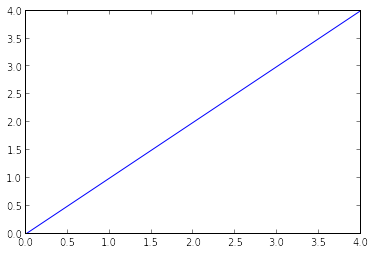I am currently working in Linux, and have stored the dataset in the following location:

### Importing libraries and the data set:

Following are the libraries we will use during this tutorial:

• numpy
• matplotlib
• pandas

Please note that you do not need to import matplotlib and numpy because of Pylab environment. I have still kept them in the code, in case you use the code in a different environment.

After importing the library, you read the dataset using function read_csv(). This is how the code looks like till this stage:

import pandas as pd import numpy as np import matplotlib as plt df = pd.read_csv(“/home/kunal/Downloads/Loan_Prediction/train.csv”) #Reading the dataset in a dataframe using Pandas

### Quick Data Exploration

Once you have read the dataset, you can have a look at few top rows by using the function head()This should print 10 rows. Alternately, you can also look at more rows by printing the dataset.

Next, you can look at summary of numerical fields by using describe() function

df.describe()describe() function would provide count, mean, standard deviation (std), min, quartiles and max in its output

Here are a few inferences, you can draw by looking at the output of describe() function:

1. LoanAmount has (614 – 592) 22 missing values.
2. Loan_Amount_Term has (614 – 600) 14 missing values.
3. Credit_History has (614 – 564) 50 missing values.
4. We can also look that about 84% applicants have a credit_history. How? The mean of Credit_History field is 0.84 (Remember, Credit_History has value 1 for those who have a credit history and 0 otherwise)
5. The ApplicantIncome distribution seems to be in line with expectation. Same with CoapplicantIncome

Please note that we can get an idea of a possible skew in the data by comparing the mean to the median, i.e. the 50% figure.

For the non-numerical values (e.g. Property_Area, Credit_History etc.), we can look at frequency distribution to understand whether they make sense or not. The frequency table can be printed by following command:

df[‘Property_Area’].value_counts()

Similarly, we can look at unique values of port of credit history. Note that dfname[‘column_name’] is a basic indexing technique to acess a particular column of the dataframe. It can be a list of columns as well. For more information, refer to the “10 Minutes to Pandas” resource shared above.

### Distribution analysis

Now that we are familiar with basic data characteristics, let us study distribution of various variables. Let us start with numeric variables – namely ApplicantIncome and LoanAmount

Lets start by plotting the histogram of ApplicantIncome using the following commands:

df[‘ApplicantIncome’].hist(bins=50)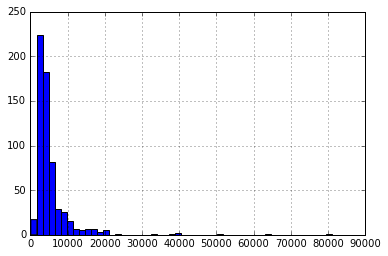Here we observe that there are few extreme values. This is also the reason why 50 bins are required to depict the distribution clearly.

Next, we look at box plots to understand the distributions. Box plot for fare can be plotted by:

df.boxplot(column=’ApplicantIncome’)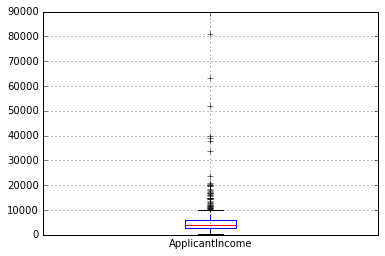This confirms the presence of a lot of outliers/extreme values. This can be attributed to the income disparity in the society. Part of this can be driven by the fact that we are looking at people with different education levels. Let us segregate them by Education:

df.boxplot(column=’ApplicantIncome’, by = ‘Education’)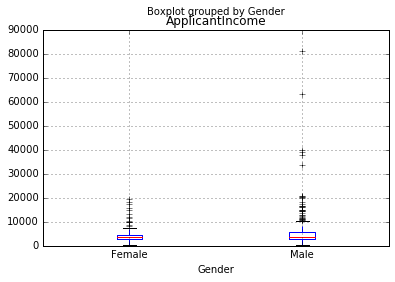We can see that there is no substantial different between the mean income of graduate and non-graduates. But there are a higher number of graduates with very high incomes, which are appearing to be the outliers.

Now, Let’s look at the histogram and boxplot of LoanAmount using the following command:

df[‘LoanAmount’].hist(bins=50)
`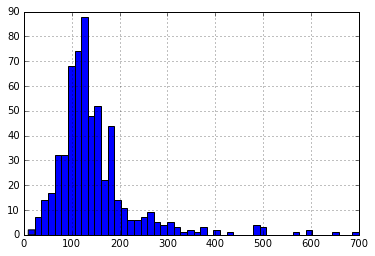`
df.boxplot(column=’LoanAmount’)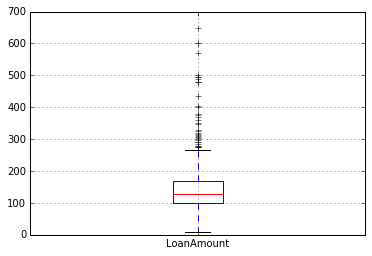Again, there are some extreme values. Clearly, both ApplicantIncome and LoanAmount require some amount of data munging. LoanAmount has missing and well as extreme values values, while ApplicantIncome has a few extreme values, which demand deeper understanding. We will take this up in coming sections.

### Categorical variable analysis

Now that we understand distributions for ApplicantIncome and LoanIncome, let us understand categorical variables in more details. We will use Excel style pivot table and cross-tabulation. For instance, let us look at the chances of getting a loan based on credit history. This can be achieved in MS Excel using a pivot table as:Note: here loan status has been coded as 1 for Yes and 0 for No. So the mean represents the probability of getting loan.

Now we will look at the steps required to generate a similar insight using Python. Please refer to this article for getting a hang of the different data manipulation techniques in Pandas.

temp1 = df[‘Credit_History’].value_counts(ascending=True)
temp2 = df.pivot_table(values=’Loan_Status’,index=[‘Credit_History’],aggfunc=lambda x: x.map({‘Y’:1,’N’:0}).mean())
print ‘Frequency Table for Credit History:’
print temp1
print ‘\nProbility of getting loan for each Credit History class:’
print temp2Now we can observe that we get a similar pivot_table like the MS Excel one. This can be plotted as a bar chart using the “matplotlib” library with following code:

import matplotlib.pyplot as plt
fig = plt.figure(figsize=(8,4))
ax1.set_xlabel(‘Credit_History’)
ax1.set_ylabel(‘Count of Applicants’)
ax1.set_title(“Applicants by Credit_History”)
temp1.plot(kind=’bar’)
temp2.plot(kind = ‘bar’)
ax2.set_xlabel(‘Credit_History’)
ax2.set_ylabel(‘Probability of getting loan’)
ax2.set_title(“Probability of getting loan by credit history”)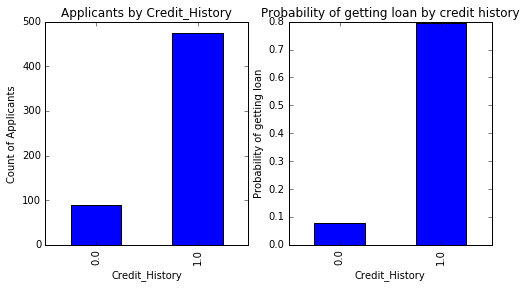This shows that the chances of getting a loan are eight-fold if the applicant has a valid credit history. You can plot similar graphs by Married, Self-Employed, Property_Area, etc.Alternately, these two plots can also be visualized by combining them in a stacked chart::

temp3 = pd.crosstab(df[‘Credit_History’], df[‘Loan_Status’])
temp3.plot(kind=’bar’, stacked=True, color=[‘red’,’blue’], grid=False)You can also add gender into the mix (similar to the pivot table in Excel):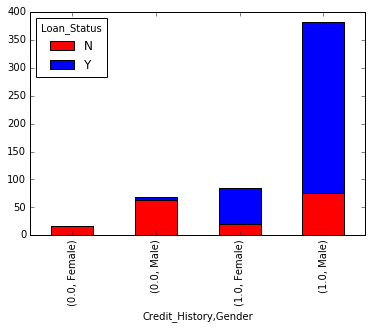If you have not realized already, we have just created two basic classification algorithms here, one based on credit history, while other on 2 categorical variables (including gender).

We just saw how we can do exploratory analysis in Python using Pandas. I hope your love for pandas (the animal) would have increased by now – given the amount of help, the library can provide you in analyzing datasets.